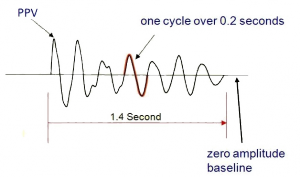# FrequencyFrequency is used to describe the oscillating nature of the vibration. We tend to talk about a vibration as being composed of cycles. Frequency is mathematically determined by taking the inverse of the time it takes to complete one cycle. This value is commonly expressed in Hertz (cycles per second). The red highlight in the image represents one cycle that lasts about 0.2 seconds. The frequency of this cycle would be 1 divided by 0.2 seconds which equals 5 Hertz (Hz). Frequency plays an important role in how individuals perceive ground vibration and how structures react to ground vibration.